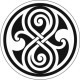# LOG#122. Basic Neutrinology(VII).

The observed mass and mixing both in the neutrino and quark cases could be evidence for some interfamily hierarchy hinting that the lepton and quark sectors were, indeed, a result of the existence of a new quantum number related to “family”. We could name this family symmetry as$U(1)_F$. It was speculated by people like Froggatt long ago. The actual intrafamily hierarchy, i.e., the fact that$m_u>>m_d$ in the quark sector, seem to require one of these symmetries to be anomalous.

A simple model with one family dependent anomalous U(1) beyond the SM was first proposed long ago to produce the given Yukawa coupling and their hierarchies, and the anomalies could be canceled by the Green-Schwarz mechanism which as by-product is able to fix the Weinberg angle as well. Several developments include the models inspired by the$E_6\times E_8$ GUT or the$E_8\times E_8$ heterotic superstring theory. The gauge structure of the model is that of the SM but enlarged by 3 abelian U(1) symmetries and their respective fields, sometimes denoted by$X,Y^{1,2}$. The first one is anomalous and family independent. Two of these fields, the non-anomalous, have specific dependencies on the 3 chiral families designed to reproduce the Yukawa hierarchies. There are right-handed neutrinos which “trigger” neutrino masses by some special types of seesaw mechanisms.

The 3 symmetries and their fields$X,Y^{1,2}$ are usually spontaneously broken at some high energy scale$M_X$ by stringy effects. It is assumed that 3 fields,$\theta_i$, with$i=1,2,3$, develop a non-null vev. These$\theta_i$ fields are singlets under the SM gauge group but not under the abelian symmetries carried by$X, Y^{1,2}$. Thus, the Yukawa couplings appear as some effective operators after the$U(1)_F$ spontaneous symmetry breaking. In the case of neutrinos, we have the mass lagrangian (at effective level):$\mathcal{L}_m\sim h_{ij}L_iH_uN_j^c\lambda^{q_i+n_j}+M_N\xi_{ij}N_i^cN_j^c\lambda^{n_i+n_j}$

and where$h_ {ij},\xi_{ij}\sim \mathcal{O}(1)$. The parameters$\lambda$ determine the mass and mixing hierarchy with the aid of some simple relationships:$\lambda=\dfrac{\langle \theta\rangle}{M_X}\sim\sin\theta_c$

and where$\theta_c$ is the Cabibblo angle. The$q_i,n_i$ are the$U(1)_F$ charges assigned to the left handed leptons L and the right handed neutrinos N. These couplings generate the following mass matrices for neutrinos:$m_\nu^D=\mbox{diag}(\lambda^{q_1},\lambda^{q_2},\lambda^{q_3})\hat{h}\mbox{diag}(\lambda^{n_1},\lambda^{n_2},\lambda^{n_3})\langle H_u\rangle$$M_\nu=\mbox{diag}(\lambda^{n_1},\lambda^{n_2},\lambda^{n_3})\hat{\xi}\mbox{diag}(\lambda^{n_1},\lambda^{n_2},\lambda^{n_3})M_N$

From these matrices, the associated seesaw mechanism gives the formula for light neutrinos:$m_\nu\approx \dfrac{\langle H_u\rangle^2}{M_X}\mbox{diag}(\lambda^{q_1},\lambda^{q_2},\lambda^{q_3})\hat{h}\hat{\xi}^{-1}\hat{h}^T\mbox{diag}(\lambda^{q_1},\lambda^{q_2},\lambda^{q_3})$

The neutrino mass mixing matrix depends only on the charges we assign to the LH neutrinos due to cancelation of RH neutrino charges and the seesaw mechanism. There is freedom in the assignment of the charges$q_i$. If the charges of the second and the third generation of leptons are equal (i.e., if$q_2=q_3$), then one is lead to a mass matrix with the following structure (or “texture”):$m_\nu\sim \begin{pmatrix}\lambda^6 & \lambda^3 & \lambda^3\\ \lambda^3 & a & b\\ \lambda^3 & b & c\end{pmatrix}$

and where$a,b,c\sim \mathcal{O}(1)$. This matrix can be diagonalized in a straightforward fashion by a large$\nu_2-\nu_3$ rotation. It is consistent (more or less), with a large$\mu-\tau$ mixing. In this theory or model, the explanation of the large neutrino mixing angles is reduced to a theory of prefactors in front of powers of the parameters$\lambda$, related with the vev after the family group spontaneous symmetry breaking!

View ratings
Rate this article

### Comments

#### LOG#122. Basic Neutrinology(VII). — 2 Comments

1.John on said:

Hola!! Podrías dejar alguna bibliografía sobre este post?? Me ha interesado especialmente!!!

Thanks!!

•amarashiki on said:

Hi. This site is yet “in construction” (It will be an upgrade from the free site http://thespectrumofriemannium.wordpress.com ). I am surprised you found it! 🙂 You will have to wait a little bit for an answer. I have some duties with this new domain and IRL at the moment. If you are patient, I can attend your petition in a few weeks. I have some posts yet in the neutrinology thread in the free site and with this domain I will not be ready until September, I guess… I wish you understand. :).